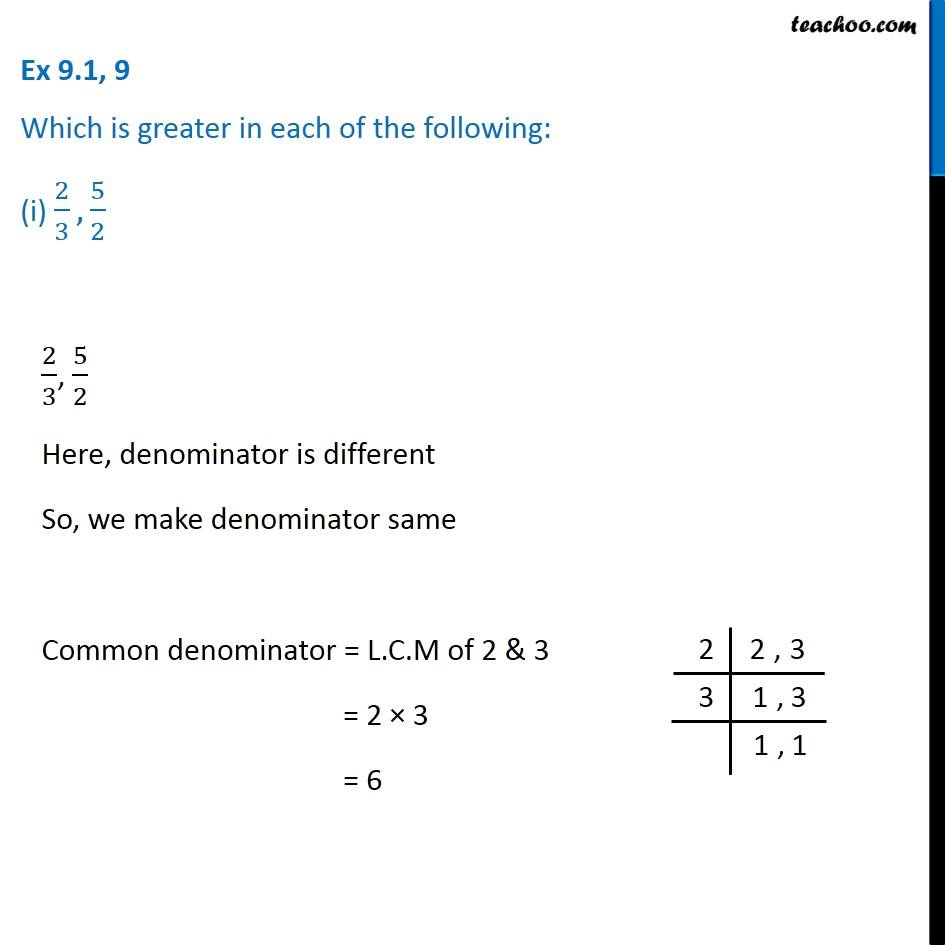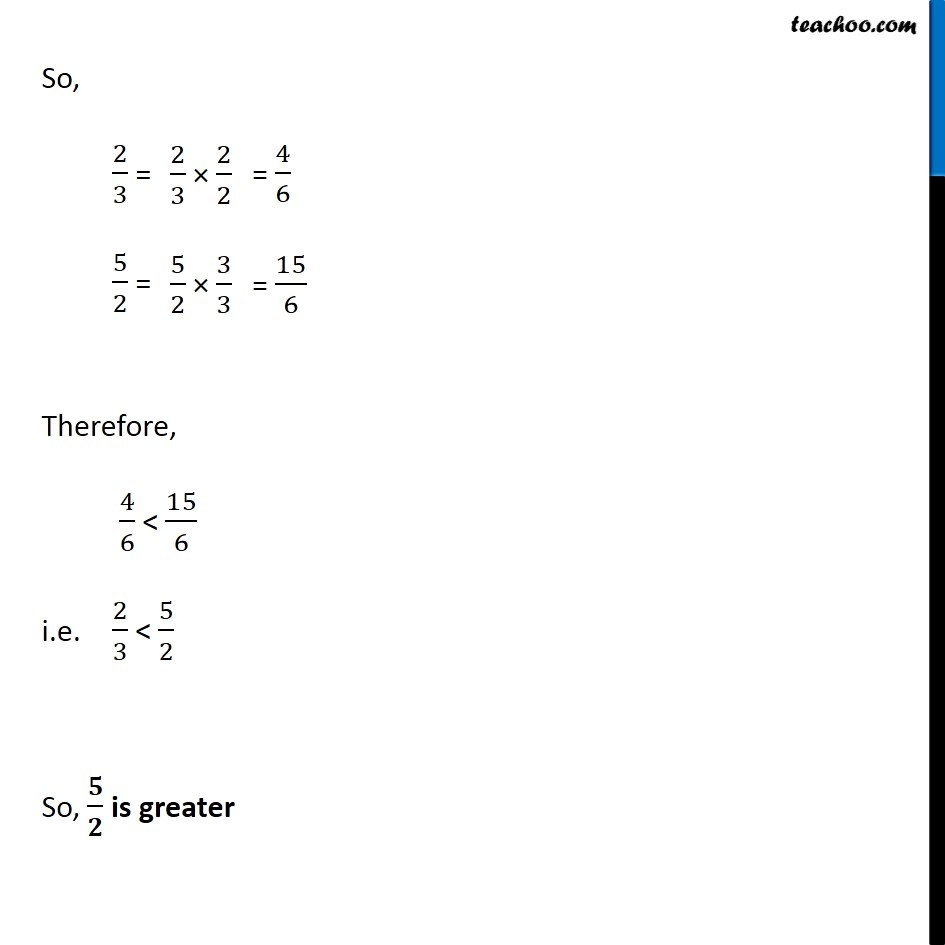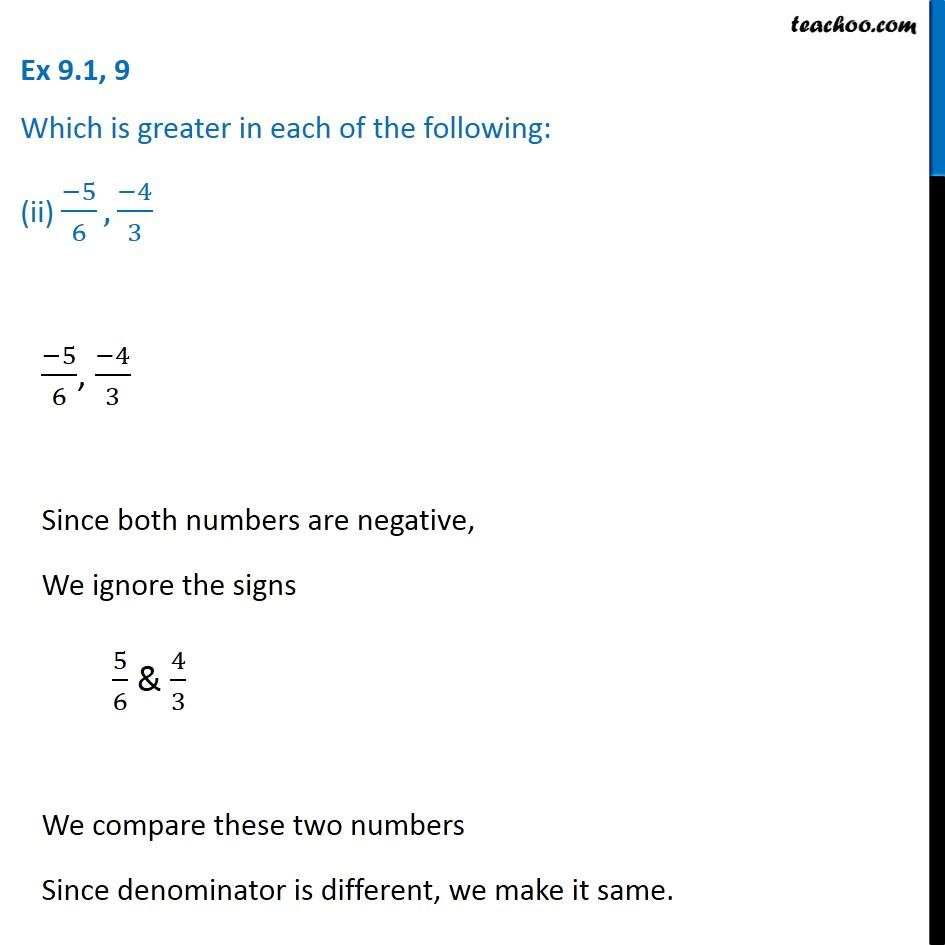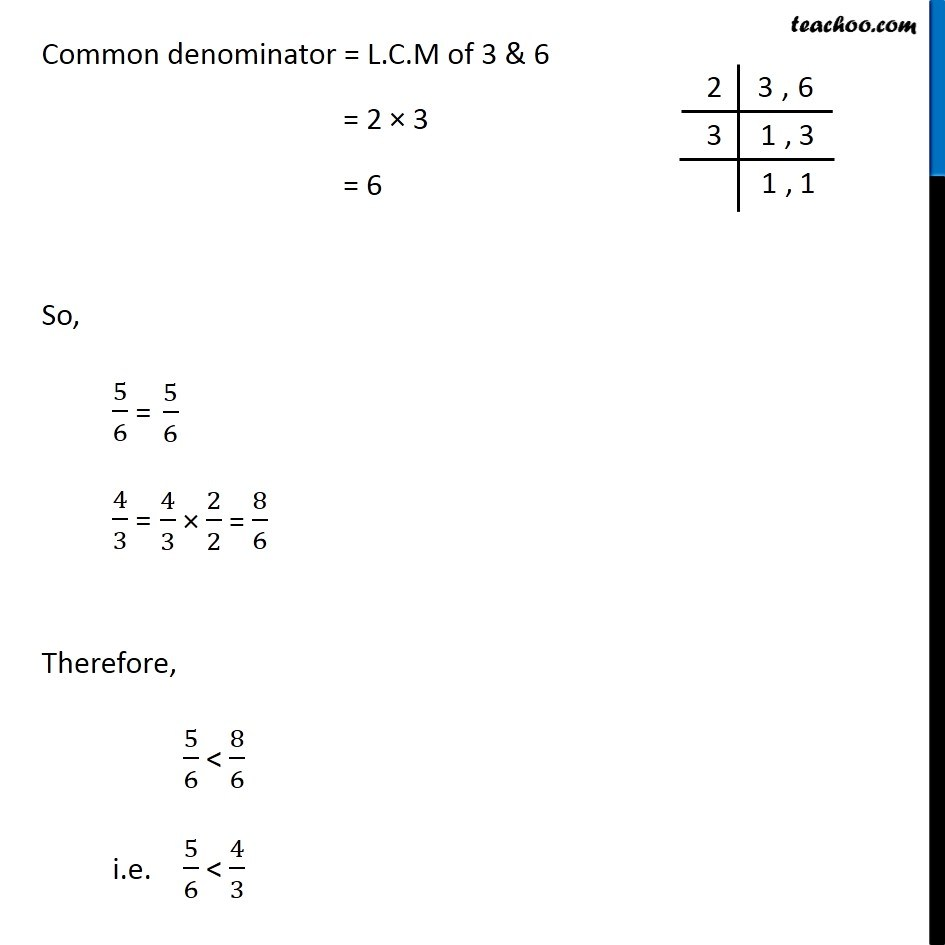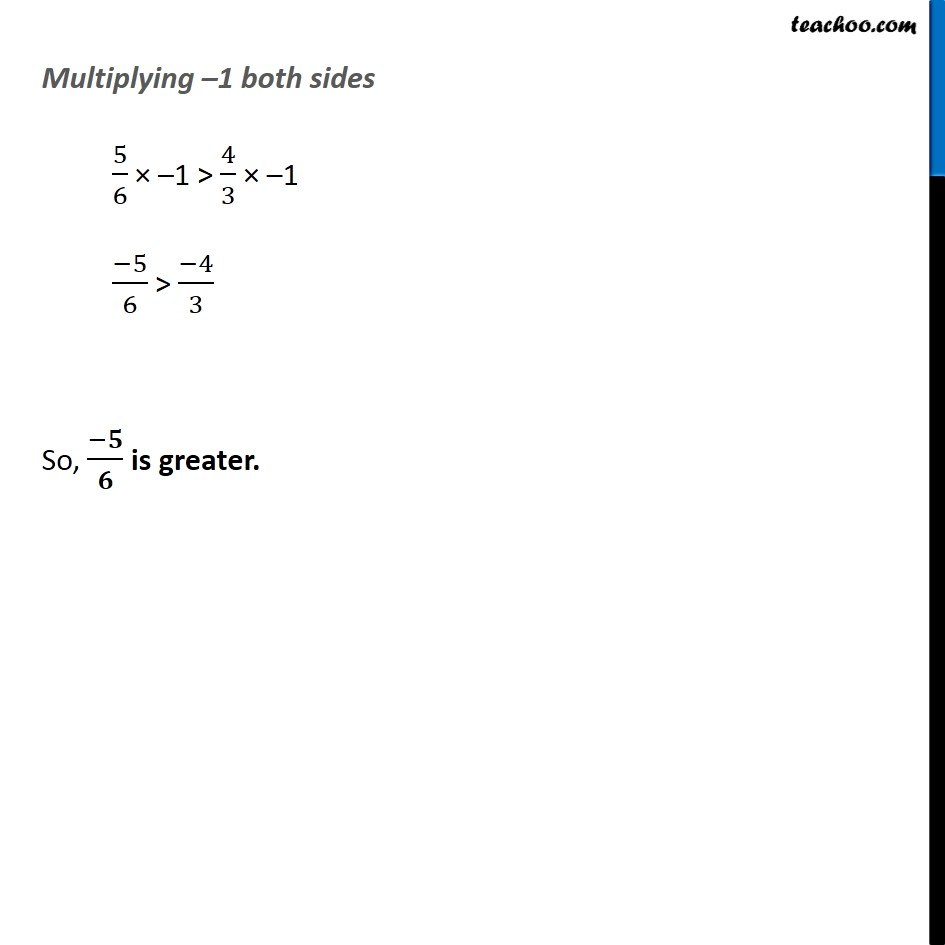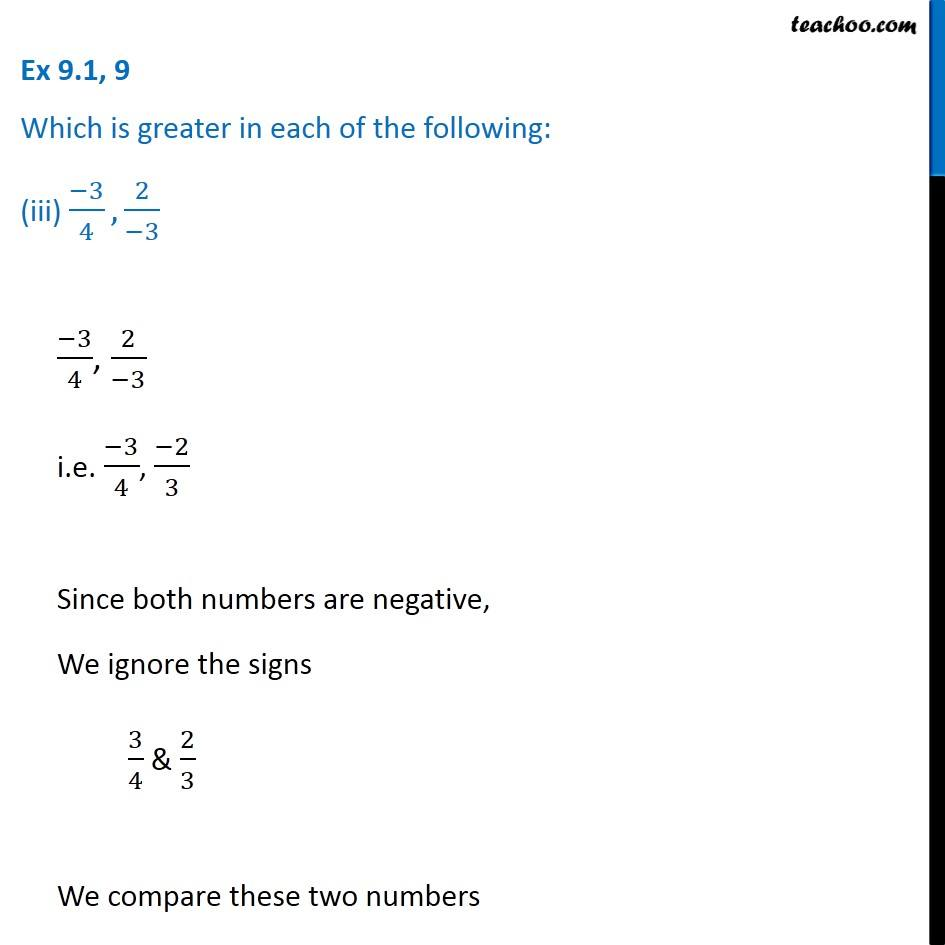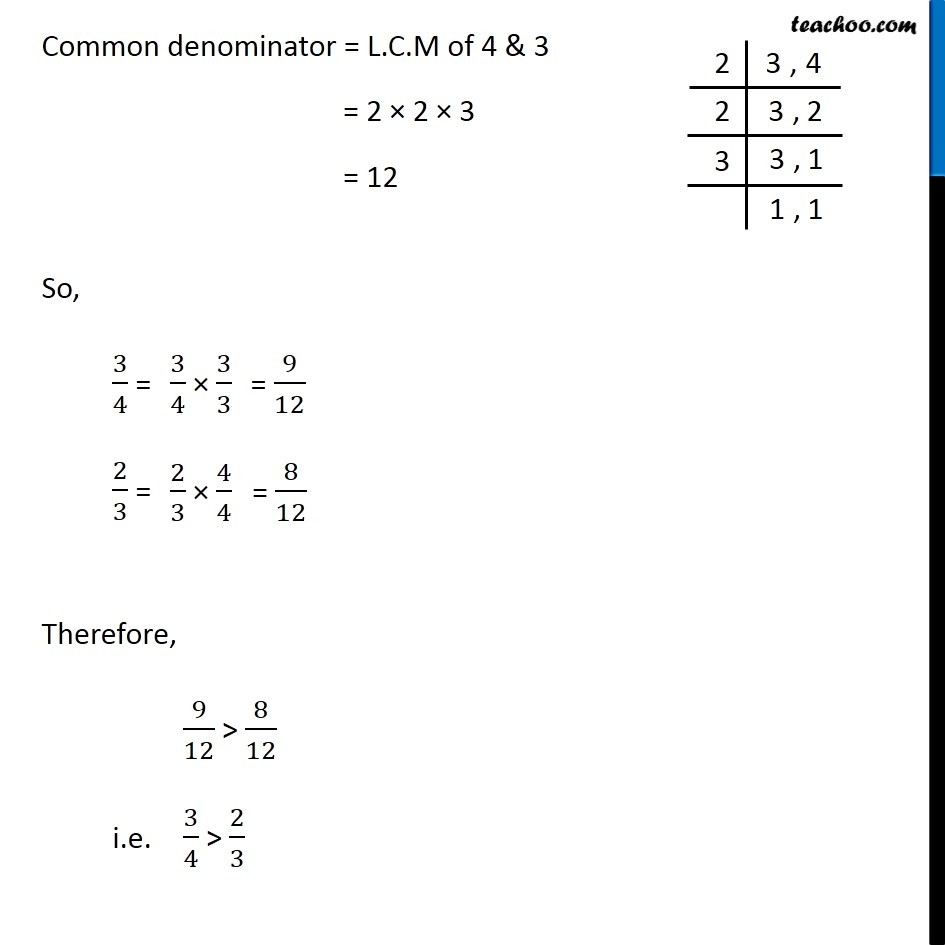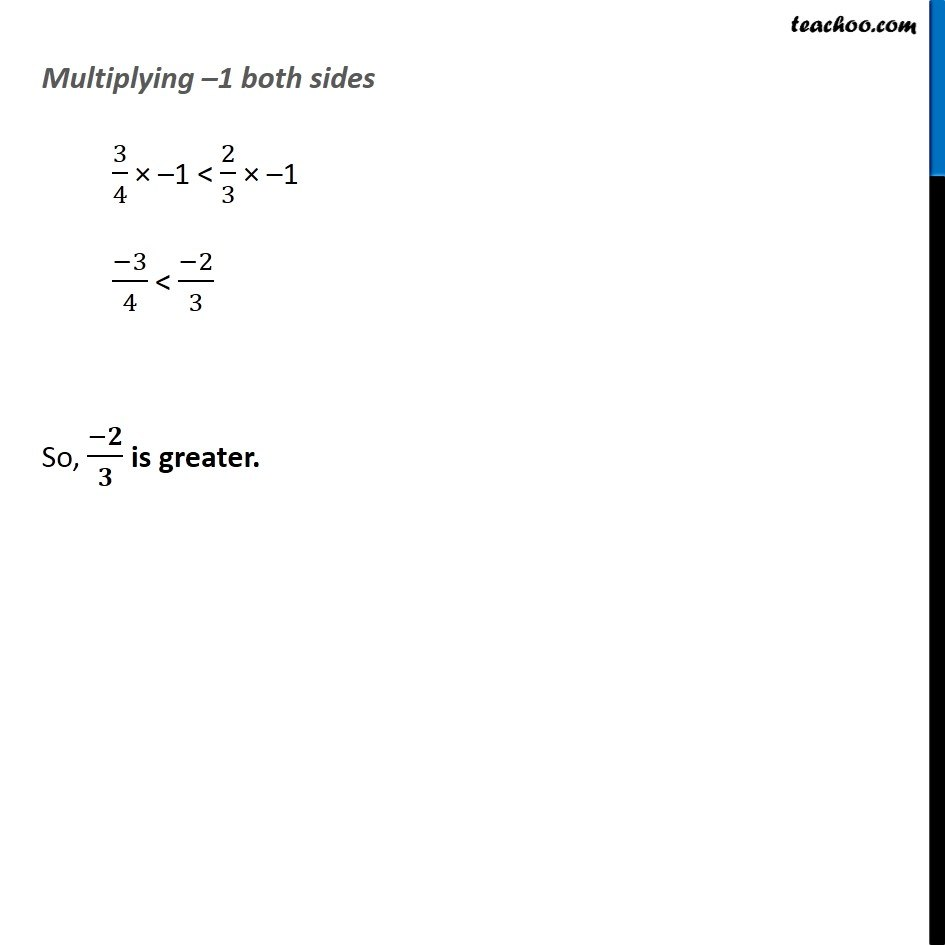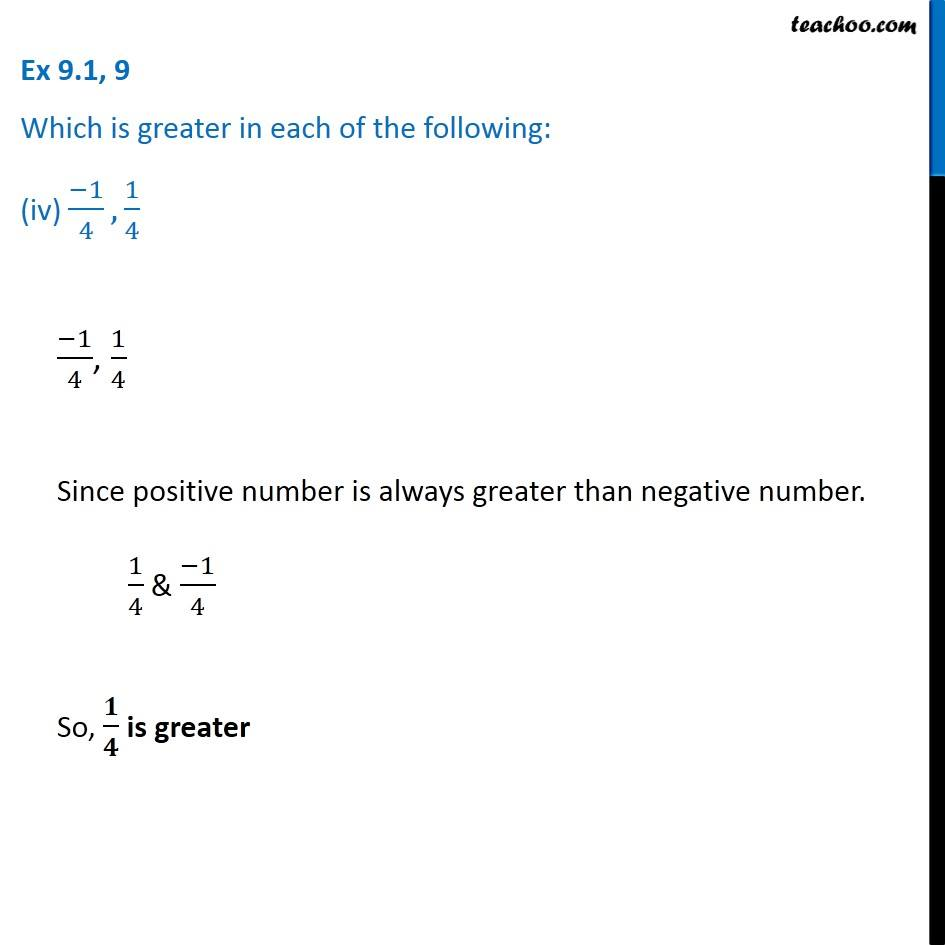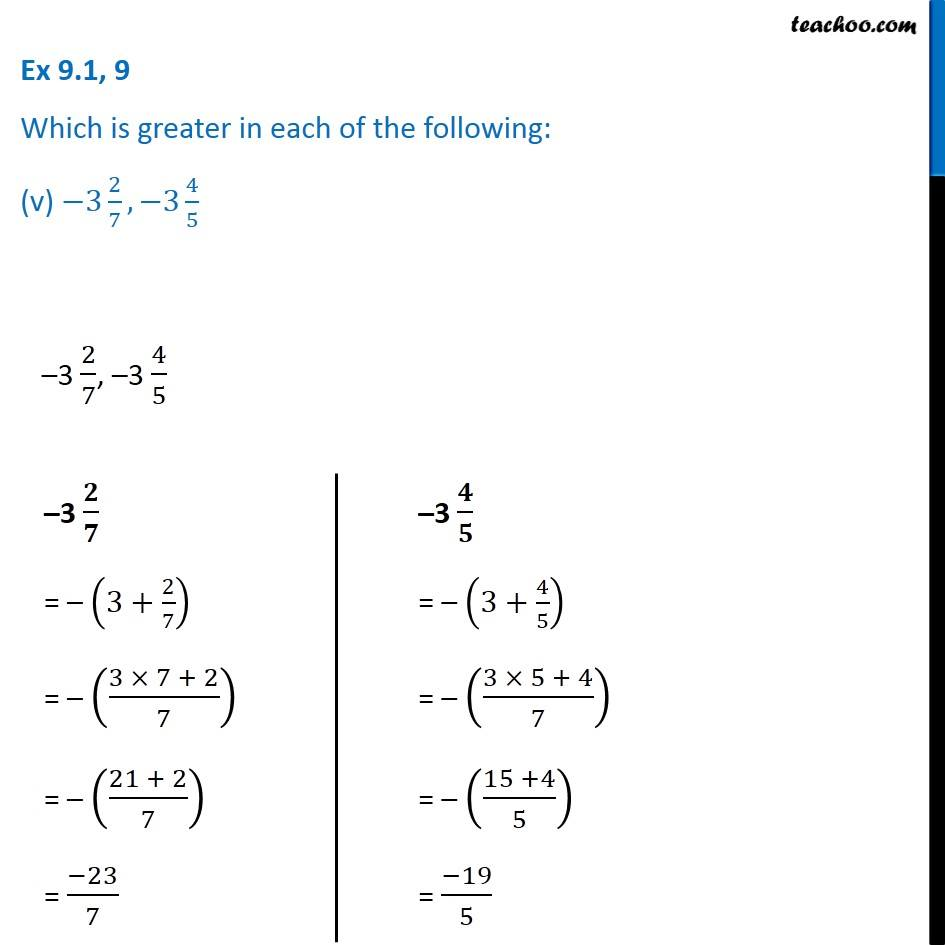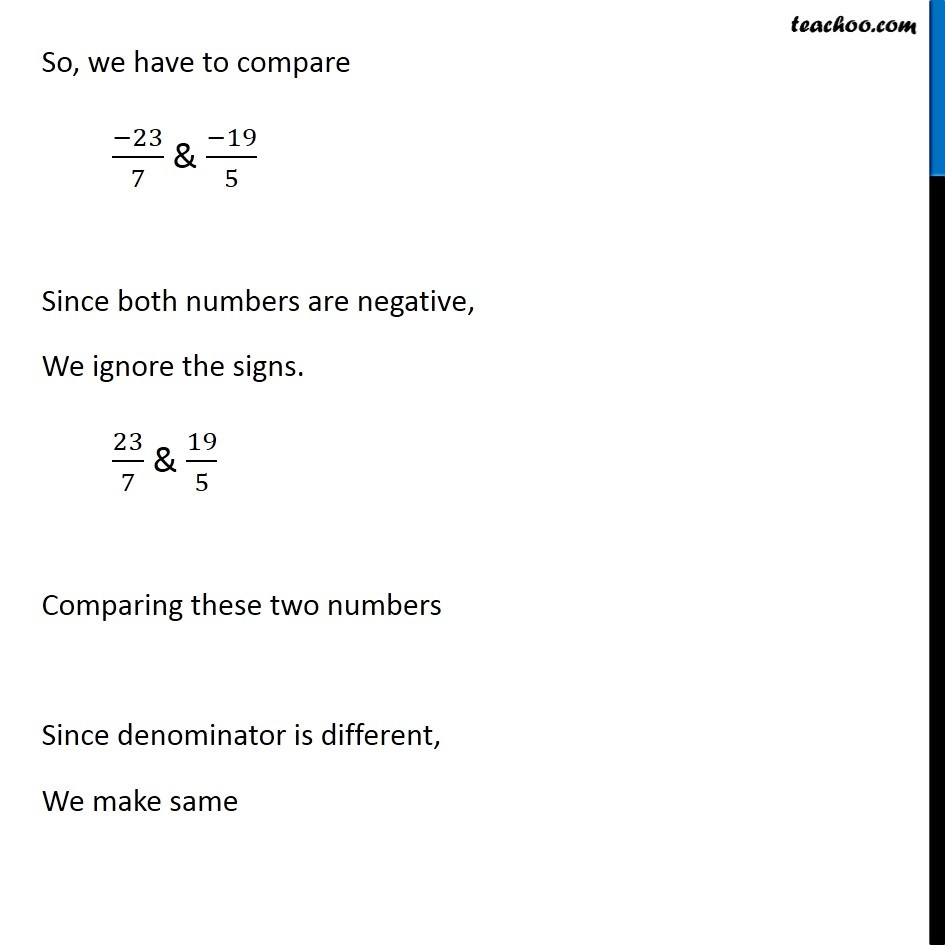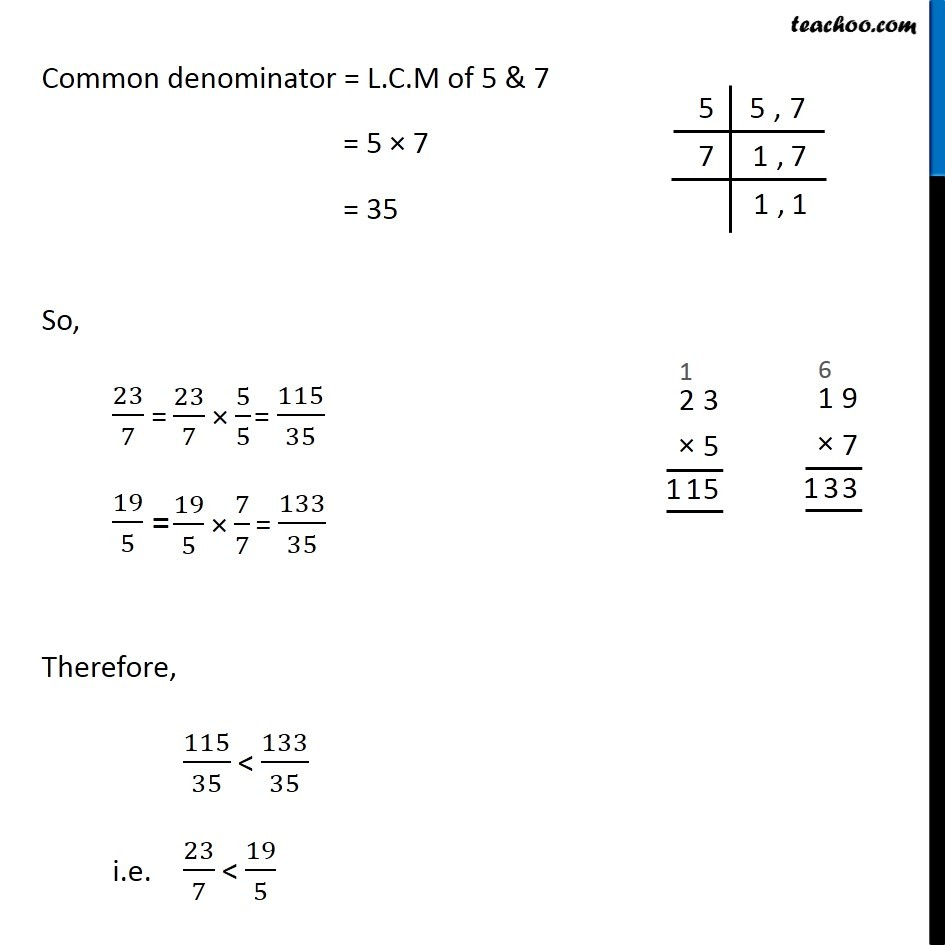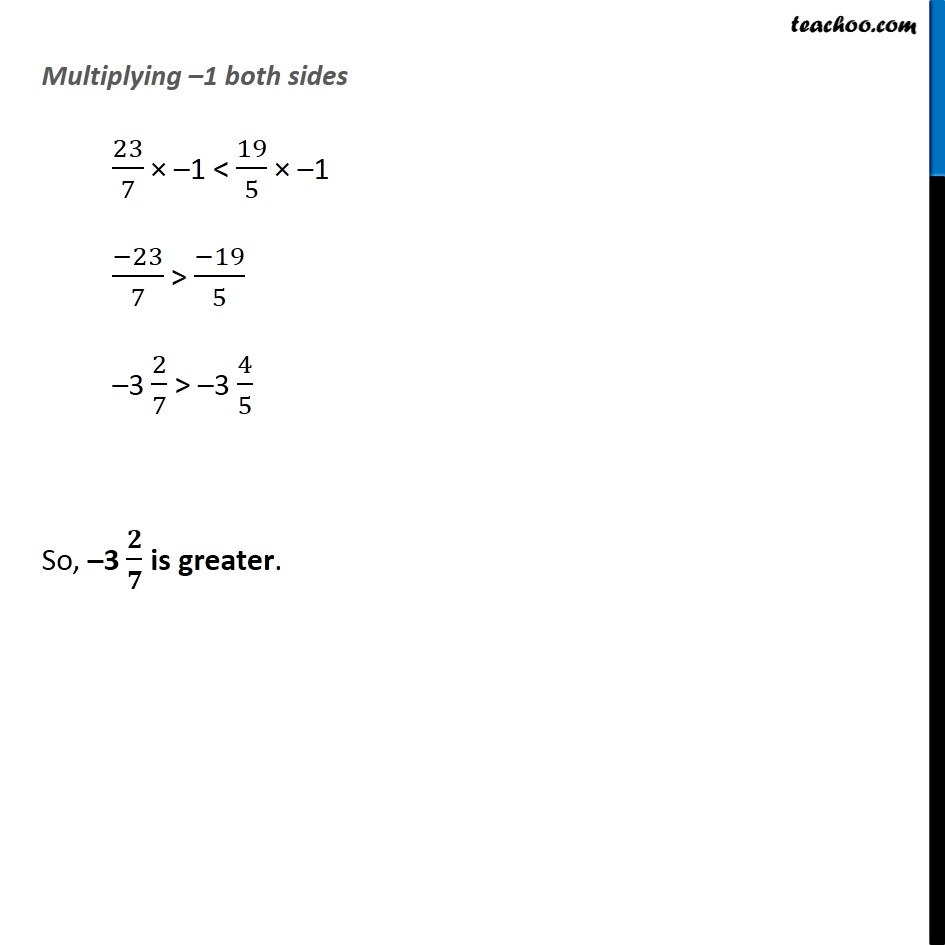Subscribe to our Youtube Channel - https://you.tube/teachoo

1. Chapter 9 Class 7 Rational Numbers
2. Serial order wise
3. Ex 9.1

Transcript

Ex 9.1, 9 Which is greater in each of the following: (i) 2/3, 5/2 2/3, 5/2 Here, denominator is different So, we make denominator same Common denominator = L.C.M of 2 & 3 = 2 × 3 = 6 So, 2/3 = 5/2 = Therefore, 4/6 < 15/6 i.e. 2/3 < 5/2 So, 𝟓/𝟐 is greater Ex 9.1, 9 Which is greater in each of the following: (ii) (−5)/6, (−4)/3 (−5)/6, (−4)/3 Since both numbers are negative, We ignore the signs 5/6 & 4/3 We compare these two numbers Since denominator is different, we make it same. Common denominator = L.C.M of 3 & 6 = 2 × 3 = 6 So, 5/6 = 4/3 = Therefore, 5/6 < 8/6 i.e. 5/6 < 4/3 Multiplying –1 both sides 5/6 × –1 > 4/3 × –1 (−5)/6 > (−4)/3 So, (−𝟓)/𝟔 is greater. Ex 9.1, 9 Which is greater in each of the following: (iii) (−3)/4, 2/(−3) (−3)/4, 2/(−3) i.e. (−3)/4, (−2)/3 Since both numbers are negative, We ignore the signs 3/4 & 2/3 We compare these two numbers Common denominator = L.C.M of 4 & 3 = 2 × 2 × 3 = 12 So, 3/4 = 2/3 = Therefore, 9/12 > 8/12 i.e. 3/4 > 2/3 Multiplying –1 both sides 3/4 × –1 < 2/3 × –1 (−3)/4 < (−2)/3 So, (−𝟐)/𝟑 is greater. (−1)/4, 1/4 Since positive number is always greater than negative number. 1/4 & (−1)/4 So, 𝟏/𝟒 is greater Ex 9.1, 9 Which is greater in each of the following: (v) −3 2/7, −3 4/5 –3 2/7, –3 4/5 –3 𝟐/𝟕 = – (3+2/7) = – ((3 × 7 + 2)/7) = – ((21 + 2)/7) = (−23)/7 –3 𝟒/𝟓 = – (3+4/5) = – ((3 × 5 + 4)/7) = – ((15 +4)/5) = (−19)/5 So, we have to compare (−23)/7 & (−19)/5 Since both numbers are negative, We ignore the signs. 23/7 & 19/5 Comparing these two numbers Since denominator is different, We make same Common denominator = L.C.M of 5 & 7 = 5 × 7 = 35 So, 23/7 = 19/5 = Therefore, 115/35 < 133/35 i.e. 23/7 < 19/5 Multiplying –1 both sides 23/7 × –1 < 19/5 × –1 (−23)/7 > (−19)/5 –3 2/7 > –3 4/5 So, –3 𝟐/𝟕 is greater.

Ex 9.1